• 利用引力公式确定城市化人口的区域分布，罗志刚，，介绍了利用引力公式计算区域城市化人口分配的方法。该方法对大规模的人口跨区域流动具有较强的处理能力，对构建新的城市体系具有
• 为提高网络吞吐量、缓解交通拥塞程度，结合复杂网络理论和引力场理论研究了在交通引力场下的动态路由选择过程，定义了传输路径对数据包的引力计算公式。基于路径的引力，顾及数据包的传输路径长度、节点畅通度及介数...网络拥塞 路由策略 复杂网络
• ## 引力搜索算法

千次阅读 2020-07-03 21:10:37
最近在论文中看到有学者用改进的引力搜索算法解优化问题，有一个较好的效果，于是去了解了一下这个算法。 引力搜索算法(Gravitational Search Algorithm，GSA)是Esmat Rashedi等人在2009年提出的一种随机性启发式...
最近在论文中看到有学者用改进的引力搜索算法解优化问题，有一个较好的效果，于是去了解了一下这个算法。
引力搜索算法(Gravitational Search Algorithm，GSA)是Esmat Rashedi等人在2009年提出的一种随机性启发式搜索算法，这种算法的灵感来自于牛顿的万有引力定律与运动定律：1.任意两个质点有通过连心线方向上的力相互吸引，该引力大小与它们质量的乘积成正比与它们距离的平方成反比。2.力使物体获得加速度。
在GSA中，质点被抽象成解空间的一个解，解之间存在一个相互吸引力，这个吸引力由解的质量与两个解之间的距离确定，质点的质量被抽象成解的评估函数值。在解空间中，每个解由其他解对其的吸引力获得加速度，质量更大(评估函数值更优)所提供的加速度更大，从而使解向更优解的方向移动。
看到这里，有些小伙伴会以为GSA是个与PSO差不多的算法，是的，GSA与PSO的外层框架是一致的，但是，它们最关键的粒子移动策略却是不同的：
1.在PSO中，粒子的移动只使用两个最佳位置pbest和gbest。但是在GSA中，智能体的方向是根据所有其他智能体或部分较优的智能体获得的总力来计算的。 2.PSO使用一种内存来更新速度（由于pbest和gbest）。然而，GSA是无内存的，只有智能体的当前位置在更新过程中起作用。 3.在PSO中，更新不考虑解之间的距离，而在GSA中，力与解之间的距离成反比。 4.PSO模拟了鸟类的社会行为，而GSA的灵感来自于物理现象。
GSA的主要过程如下：
1. 确定搜索空间。 2. 随机初始化个体种群。 3. 对种群进行适应度评价。 4. 更新引力常量G，更新种群中最好的个体 best与最差的个体worst，更新个体的质量。 5. 计算每个个体在不同方向上的总引力。 6. 计算个体的加速度，基于此更新个体的速度。 7. 根据速度更新个体在解空间中的位置。 8. 重复步骤3-7直到达到停止标准。
算法流程：具体的个体属性更新公式与引力计算公式建议参考 GSA: A Gravitational Search Algorithm这篇文章。
例： min z = x1 ** 2 + x2 ** 2 s.t. -10 <= x1 <= 10 , -10 <= x2 <= 10
# Gravitational Search Algorithm
import numpy as np
import random as rd
from math import exp, sqrt

'''min z = X1 ** 2 + X2 ** 2
s.t.   -10 <= x1 <= 10
-10 <= X2 <= 10   '''

def init(n):
position, velocity = [], []
for i in range(n):
X1 = rd.uniform(-10, 10)
X2 = rd.uniform(-10, 10)
V1 = rd.uniform(-3, 3)
V2 = rd.uniform(-3, 3)
position.append([X1, X2])
velocity.append([V1, V2])
return position, velocity

def objFuntion(x1, x2):
return x1 ** 2 + x2 ** 2

def fitnessEva(position):
fitness = []
for i in range(len(position)):
fitness.append(objFuntion(position[i], position[i]))
return fitness

def findBestAndWorst(position):
return min(fitnessEva(position)), max(fitnessEva(position))

def calculateMass(fitness):
mass = []
Mass = []
for i in range(len(fitness)):
mass.append((fitness[i] - max(fitness)) / (min(fitness) - max(fitness)))
for i in range(len(mass)):
Mass.append(mass[i] / sum(mass))
return Mass

def calculateAcceleration(position, Mass, G, topK):
acceleration = []
Fi0, Fi1 = 0, 0

for i in range(len(position)):
for j in range(len(position)):
if i != j and j in topK:
Fi0 += rd.random() * G * ((Mass[i] * Mass[j]) / (calculateDistance(position[i], position[j]) + r)) * (
position[j] - position[i])
Fi1 += rd.random() * G * ((Mass[i] * Mass[j]) / (calculateDistance(position[i], position[j]) + r)) * (
position[j] - position[i])
if Mass[i] != 0:
acceleration.append([Fi0 / Mass[i] / 10, Fi1 / Mass[i] / 10])   #这里除10是为了避免粒子的加速度过大
else:
acceleration.append([10, 10])
Fi0 = 0
Fi1 = 0
return acceleration

def findTopK(fitness, K):
topK = []
dic = {}
for i in range(len(fitness)):
dic[i] = fitness[i]
fitness.sort()
for i in range(K):
topK.append(list(dic.keys())[list(dic.values()).index(fitness[i])])

def updateVelocityAndPosition(acceleration, position, velocity):
for i in range(len(velocity)):
velocity[i] = rd.random() * velocity[i] + acceleration[i]
velocity[i] = rd.random() * velocity[i] + acceleration[i]

position[i] = position[i] + velocity[i]
position[i] = position[i] + velocity[i]

def calculateDistance(p1,p2):
return sqrt((p1 - p2) ** 2 + (p1 - p2) ** 2)

def checkPosition(position):
for i in range(len(position)):
if position[i] < -10:
position[i] = -10
elif position[i] > 10:
position[i] = 10
if position[i] < -10:
position[i] = -10
elif position[i] > 10:
position[i] = 10

if __name__ == '__main__':
G = 100
r = 1
K = 50

iterx, maxIterx = 0, 50
position, velocity = init(50)
while iterx < maxIterx:
fitness = fitnessEva(position)               #适应性评估
G = G * exp(-20 * iterx / maxIterx)          #更新引力常量
Mass = calculateMass(fitness)                #更新粒子质量
topK = findTopK(fitness, K)                  #找出适应度更优的前K个粒子
acceleration = calculateAcceleration(position, Mass, G, topK)          #计算粒子加速度
updateVelocityAndPosition(acceleration, position, velocity)            #根据加速度更新速度与位置
checkPosition(position)                                                #检查粒子是否冲出了解空间
iterx += 1
K = K - iterx                                #更新K值
print(min(fitnessEva(position)))
print(position[fitnessEva(position).index(min(fitnessEva(position)))])

参考文献： Rashedi E, Nezamabadi-pour H, Saryazdi S. GSA:a gravitational search algorithm[J].Information Science, 2009, 179 (13) :2232-2248.
展开全文算法 python
• 利用Landau-Lifshitz的能量-动量张量表述和四极矩公式对双星模型下的致密天体引力辐射源进行了讨论、计算了几种典型的致密双星系统的引力辐射（如PRSl913+16双星、PSRJ0737-3039A/B双脉冲星和双黑洞系统），并将计算...
• 参数说明： M:地球总质量M:地球总质量M:地球总质量 dm:地球上的质量微元dm:地球上的质量微元dm:地球上的质量微元 ...若外有引力做工(从无穷远移动到半径p处)为:A=∫∞p−Gdm∗mp2=Gdm∗m′p若外有引力做工(从无穷远移.参数说明：

M

:

地

球

总

质

量

M:地球总质量

d

m

:

地

球

上

的

质

量

微

元

dm:地球上的质量微元

m

′

:

地

球

外

部

空

间

上

的

一

个

质

点

m':地球外部空间上的一个质点

p

:

m

与

d

m

之

间

的

距

离

p:m与dm之间的距离

万有引力大小为：

f

=

G

d

m

∗

m

′

p

2

f=\frac{Gdm*m'}{p^2}

引力位函数

若

外

有

引

力

做

工

(

从

无

穷

远

移

动

到

半

径

p

处

)

为

:

A

=

∫

∞

p

−

G

d

m

∗

m

p

2

=

G

d

m

∗

m

′

p

若外有引力做工(从无穷远移动到半径p处)为:A=\int_\infty^p-\frac{Gdm*m}{p^2}=\frac{Gdm*m'}{p}

根据能量守恒，引力做工必然等于

m

′

m'

减少的位能（势能），若单位质量的点(从无穷远移动到半径p处)，势能必然减少，将减少量定义为位函数:

d

V

=

G

d

m

p

dV=\frac{Gdm}{p}

地球外部空间的调和函数
地球产生的各质量微元函数之和为：

V

=

∫

M

d

V

=

G

∫

M

d

m

p

（

公

式

1

）

V=\int_MdV=G\int_M\frac{dm}{p}（公式1）

又因为：

▽

V

=

∂

2

V

∂

x

2

+

∂

2

V

∂

y

2

+

∂

2

V

∂

z

2

=

0

(

证

略

)

\bigtriangledown V=\frac{\partial^2V}{\partial x^2}+\frac{\partial^2V}{\partial y^2}+\frac{\partial^2V}{\partial z^2}=0(证略)

所以

V

V

是在地球质量M的外部空间上是调和函数
勒让德多项式的生成函数参数说明：

m

′

：

的

球

坐

标

为

(

r

,

θ

,

λ

)

m'：的球坐标为(r,\theta,\lambda)

d

m

：

的

球

坐

标

为

(

r

,

θ

′

,

λ

′

)

dm：的球坐标为(r,\theta',\lambda')

向

量

R

与

r

夹

角

为

φ

向量R与r夹角为\varphi

m

0

′

是

m

′

在

球

面

上

的

投

影

m0'是m'在球面上的投影

在,

o

,

d

m

,

m

′

o,dm,m'

构成的三角形中，根据余弦定理,可以得到：

p

2

=

r

2

+

R

2

−

2

R

r

c

o

s

φ

=

r

2

(

1

−

2

a

x

+

a

2

)

(

a

=

R

r

,

x

=

c

o

s

φ

)

p^2=r^2+R^2-2Rrcos\varphi=r^2(1-2ax+a^2)(a=\frac{R}{r},x=cos\varphi)

再开平方，取倒数得到：

1

p

=

1

r

(

1

−

2

a

x

+

a

2

)

−

1

2

（

公

式

2

）

\frac{1}{p}=\frac{1}{r}(1-2ax+a^2)^{-\frac{1}{2}}（公式2）

又因为：

(

1

−

2

a

x

+

a

2

)

−

1

2

=

∑

n

=

0

∞

P

n

(

x

)

a

n

(

勒

让

德

多

项

式

的

生

成

函

数

)

（

公

式

3

）

(1-2ax+a^2)^{-\frac{1}{2}}=\sum_{n=0}^\infty P_n(x)a^n(勒让德多项式的生成函数)（公式3）

所以

(

1

−

2

a

x

+

a

2

)

−

1

2

(1-2ax+a^2)^{-\frac{1}{2}}

为勒让德多项式的生成函数
球函数的加法公式根据球面的三角余弦定理有:

c

o

s

φ

=

c

o

s

θ

c

o

s

θ

′

+

s

i

n

θ

s

i

n

θ

′

c

o

s

(

λ

−

λ

′

)

cos\varphi=cos\theta cos\theta'+sin\theta sin\theta'cos(\lambda-\lambda')

带入

P

n

(

c

o

s

φ

)

=

∑

k

=

0

n

2

(

n

−

k

)

!

(

1

+

δ

k

)

(

n

+

k

)

!

P

n

k

(

c

o

s

θ

)

P

n

k

(

c

o

s

θ

′

)

c

o

s

k

λ

′

+

P

n

k

c

o

s

θ

s

i

n

(

k

λ

)

P

n

k

(

c

o

s

θ

′

)

(

s

i

n

k

λ

′

)

(

公

式

4

)

P_n(cos\varphi)=\sum_{k=0}^n\frac{2(n-k)!}{(1+\delta_k)(n+k)!}P_n^k(cos\theta)P_n^k(cos\theta')cosk\lambda'+P_n^kcos\theta sin(k\lambda) P_n^k(cos\theta')(sink\lambda') (公式4)

利用德让勒多项式求解V（调和函数）
将勒让德多项式的生成函数（公式(3)），将带入公式（2），再将公式2带入地球外部空间的调和函数公式（1）。 得到:

R

e

:

旋

转

椭

圆

球

的

长

半

径

R_e:旋转椭圆球的长半径

u

=

G

M

:

地

球

引

力

常

数

u=GM:地球引力常数

δ

k

=

{

1

k

=

0

0

k

≠

1

\delta_k= \begin{cases} 1&k=0\\ 0&k\neq1\\ \end{cases}

V

=

G

r

∑

n

=

0

∞

(

R

e

r

)

n

∫

M

(

R

R

e

)

n

P

n

(

c

o

s

φ

)

d

m

V=\frac{G}{r}\sum_{n=0}^\infty(\frac{R_e}{r})^n\int_M(\frac{R}{R_e})^nP_n(cos\varphi)dm

将公式（4）带入: 得到:

V

=

u

r

∑

n

=

0

∞

(

R

e

r

)

n

∑

k

=

0

n

(

C

n

k

c

o

s

k

λ

+

S

n

k

s

i

n

k

λ

)

P

n

k

(

c

o

s

θ

)

V=\frac{u}{r}\sum_{n=0}^\infty(\frac{R_e}{r})^n\sum_{k=0}^n(C_n^kcosk\lambda+S_n^ksink\lambda)P_n^k(cos\theta)

C

n

k

=

2

(

n

−

k

)

!

M

(

1

+

δ

k

)

(

n

+

k

)

!

∫

M

(

R

R

e

)

n

P

n

k

(

c

o

s

θ

′

)

c

o

s

k

λ

′

d

m

C_n^k=\frac{2(n-k)!}{M(1+\delta_k)(n+k)!}\int_M(\frac{R}{R_e})^nP_n^k(cos\theta')cosk\lambda'dm

S

n

k

=

2

(

n

−

k

)

!

M

(

1

+

δ

k

)

(

n

+

k

)

!

∫

M

(

R

R

e

)

n

P

n

k

(

c

o

s

θ

′

)

s

i

n

k

λ

′

d

m

S_n^k=\frac{2(n-k)!}{M(1+\delta_k)(n+k)!}\int_M(\frac{R}{R_e})^nP_n^k(cos\theta')sink\lambda'dm

已知直角坐标系到球体坐标系的转换关系如下：

{

x

=

R

s

i

n

θ

′

c

o

s

λ

′

y

=

R

s

i

n

θ

′

s

i

n

λ

′

z

=

R

c

o

s

θ

′

\begin{cases} x=Rsin\theta'cos\lambda'\\ y=Rsin\theta'sin\lambda'\\ z=Rcos\theta'\\ \end{cases}

刚体的张量定义如下:刚体的惯性张量及其物理意义

I

=

[

I

x

−

I

x

y

−

I

x

z

−

I

x

y

I

y

−

I

y

z

−

I

x

z

−

I

y

z

I

x

z

]

=

∫

M

[

y

2

+

z

2

−

x

y

−

x

z

−

x

y

x

2

+

z

2

−

y

z

−

x

z

−

y

z

x

2

+

y

2

]

d

m

I=\left[\begin{matrix} I_{x}&-I_{xy}&-I_{xz}\\ -I_{xy}&I_{y}&-I_{yz}\\ -I_{xz}&-I_{yz}&I_{xz}\\ \end{matrix}\right]=\int_M\left[\begin{matrix} y^2+z^2&-xy&-xz\\ -xy&x^2+z^2&-yz\\ -xz&-yz&x^2+y^2\\ \end{matrix}\right]dm

可以得到：

C

0

0

=

1

C_0^0=1

C

0

1

=

1

R

e

(

1

M

∫

M

z

d

m

)

C_0^1=\frac{1}{R_e}(\frac{1}{M}\int_Mzdm)

C

1

1

=

1

R

e

(

1

M

∫

M

x

d

m

)

C_1^1=\frac{1}{R_e}(\frac{1}{M}\int_Mxdm)

S

1

1

=

1

R

e

(

1

M

∫

M

y

d

m

)

S_1^1=\frac{1}{R_e}(\frac{1}{M}\int_Mydm)

C

2

0

=

1

M

R

e

2

(

∫

M

z

2

−

x

2

+

y

2

2

d

m

)

=

1

M

R

e

2

(

I

x

+

I

y

2

−

I

z

)

C_2^0=\frac{1}{MR_e^2}(\int_Mz^2-\frac{x^2+y^2}{2}dm)=\frac{1}{MR_e^2}(\frac{I_x+I_y}{2}-I_z)

C

2

1

=

1

M

R

e

2

∫

M

x

z

d

m

=

I

x

z

M

R

e

2

C_2^1=\frac{1}{MR_e^2}\int_Mxzdm=\frac{I_{xz}}{MR_e^2}

C

2

2

=

1

4

M

R

e

2

(

∫

M

x

2

−

y

2

d

m

)

=

I

y

−

I

x

4

M

R

e

2

C_2^2=\frac{1}{4MR_e^2}(\int_Mx^2-y^2dm)=\frac{I_{y}-I_{x}}{4MR_e^2}

S

2

1

=

1

M

R

e

2

(

∫

M

y

z

d

m

)

=

I

y

z

M

R

e

2

S_2^1=\frac{1}{MR_e^2}(\int_Myzdm)=\frac{I_{yz}}{MR_e^2}

S

2

2

=

1

4

M

R

e

2

(

∫

M

x

y

d

m

)

=

I

x

y

2

M

R

e

2

S_2^2=\frac{1}{4MR_e^2}(\int_Mxydm)=\frac{I_{xy}}{2MR_e^2}

如果定义直角坐标与地球惯性惯性主轴重合，则：

I

x

y

=

I

y

z

=

I

x

z

=

0

I_{xy}=I_{yz}=I_{xz}=0

对于实际应用时，地球坐标一边选择坐标原点与地球质心重合，oz与地球自转平行，ox轴在赤道面上且指向0度经线，这时坐标轴往往与惯性主轴不重合。 利用三角函数恒等式： 得到:

V

=

u

r

{

1

−

∑

n

=

1

∞

(

R

e

r

)

n

[

J

n

P

n

(

c

o

s

θ

)

+

∑

k

=

1

n

J

n

k

P

n

k

(

c

o

s

θ

)

c

o

s

k

(

λ

+

λ

n

k

)

]

}

V=\frac{u}{r}\{1-\sum_{n=1}^\infty(\frac{R_e}{r})^n[J_nP_n(cos\theta)+\sum_{k=1}^nJ_n^kP_n^k(cos\theta)cosk(\lambda+\lambda_n^k)]\}

J

n

=

−

C

n

0

,

J

n

k

=

(

C

n

k

)

2

+

(

S

n

k

)

2

,

λ

n

k

=

−

a

r

c

t

a

n

(

S

n

k

C

n

k

)

/

k

J_n=-C_n^0,J_n^k=\sqrt{(C_n^k)^2+(S_n^k)^2},\lambda_n^k=-arctan(\frac{S_n^k}{C_n^k})/k

J

2

=

C

2

0

=

1

M

R

e

2

(

I

x

+

I

y

2

−

I

z

)

(

动

力

扁

率

：

反

映

赤

道

与

极

轴

上

转

动

惯

量

的

差

别

)

J_2=C_2^0=\frac{1}{MR_e^2}(\frac{I_x+I_y}{2}-I_z)(动力扁率：反映赤道与极轴上转动惯量的差别)

u

r

:

球

形

地

球

引

起

的

引

力

位

\frac{u}{r}:球形地球引起的引力位

在实际应用中因为其他系数比

J

2

J_2

小三个数量级，所以只需考虑

u

r

和

J

2

\frac{u}{r}和J_2

的影响，可以将

V

V

近似看为：

V

=

u

r

[

1

−

J

2

R

e

2

2

r

2

(

3

c

o

s

2

θ

−

1

)

]

（

只

考

虑

主

谐

项

）

V=\frac{u}{r}[1-\frac{J_2R_e^2}{2r^2}(3cos^2\theta-1)]（只考虑主谐项）

卫星在惯性坐标系下的运动方程（只考虑主谐项）
已知

位

移

矢

量

：

r

=

[

x

y

z

]

T

位移矢量：r=\left[\begin{matrix} x&y&z\\ \end{matrix}\right]^T

引

力

：

f

=

[

f

x

f

y

f

z

]

T

引力：f=\left[\begin{matrix} f_x&f_y&f_z\\ \end{matrix}\right]^T

c

o

s

θ

=

z

r

cos\theta=\frac{z}{r}

对x,y,z求2阶片导，求得加速度方程为：

r

¨

=

−

u

r

3

[

I

+

3

2

J

2

(

R

e

r

)

2

(

D

−

5

(

u

r

∗

u

p

)

I

)

]

r

\ddot r=-\frac{u}{r^3}[I+\frac{3}{2}J_2(\frac{R_e}{r})^2(D-5(u_r*u_p)I)]r

其中:

D

=

d

i

a

g

(

1

1

3

)

D=diag\left(\begin{matrix} 1&1&3\\ \end{matrix}\right)

u

r

表

示

r

上

的

单

位

矢

量

u_r表示r上的单位矢量

u

p

表

示

自

转

轴

上

的

的

单

位

矢

量

u_p表示自转轴上的的单位矢量

u

r

∗

u

p

表

示

u

p

与

u

r

之

间

夹

角

的

余

弦

值

u_r*u_p 表示u_p与u_r之间夹角的余弦值


展开全文• 在知网上一搜“基于引力模型的空间格局研究”，有各省份相关的研究，也是通过GDP、人口等一切你能自圆其说的社会经济数据，利用ArcGIS来做各城市间的联系度。如： （图片来自《中原经济区城市间相互作用时空格局...
​公众号原文链接，欢迎关注基于引力模型的城市引力分析-城市经济联系图城市引力模型—制作城市经济联系图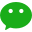https://mp.weixin.qq.com/s/EuLjbC8UbrRZh7dJHrVNvg
还是之前写的城市联系度咋实现，我稍微改了一点点，最后用Qgis调整了一下图片。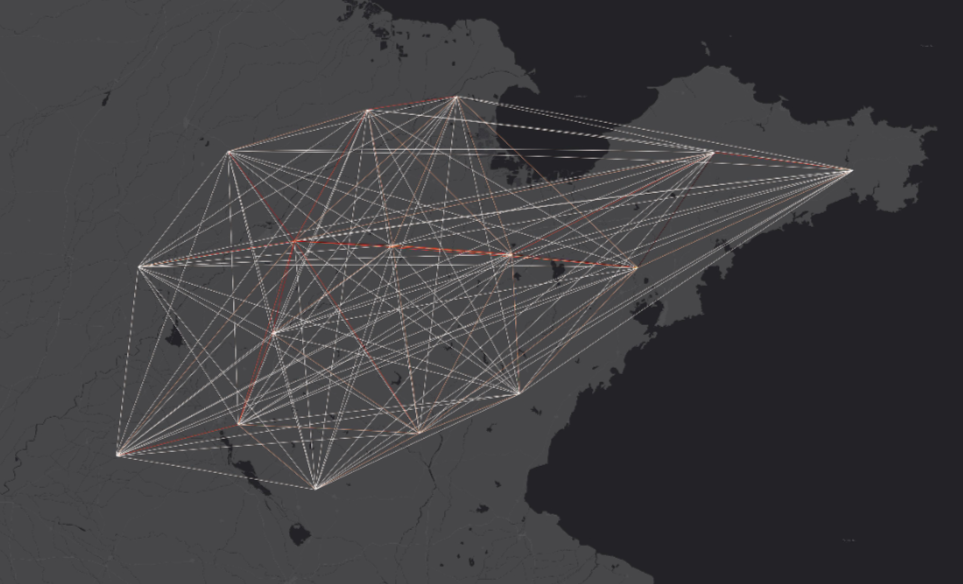一、瞎扯
在知网上一搜“基于引力模型的空间格局研究”，有各省份相关的研究，也是通过GDP、人口等一切你能自圆其说的社会经济数据，利用ArcGIS来做各城市间的联系度。如：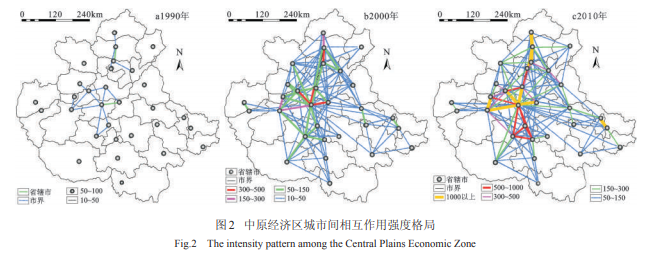（图片来自《中原经济区城市间相互作用时空格局演变研究》）
无论是用什么样的数据，原理都是基牛顿力学的万有引力公式为基础。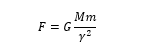Tinbergen（1962）和Poyhonen（1963）对其在经济学领域做了发展、延伸，提出了一个比较完整且简便的经济学模型—引力模型。这个模型认为两个经济体之间的单项贸易流量与它们各自的经济规模（一般用GDP来表示）成正比，与它们之间的距离成反比。
两个城市之间的引力模型公式通常可表现为：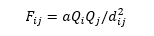其中，F为两个城市之间的引力，Q为两个城市的规模，d为两个城市之间的距离，a为常量，不影响，可取值为1。

城市规模指标Q可以是经济规模、人口规模、消费规模、进出口规模等等单项或综合；距离d可以采用实际的公路里程数，也可以采用两城市之间的空间直线距离（欧氏距离）等单项或综合。
可以采取城市综合指标处理的方式，一般采用的是求几何平均。例如选取4个指标的几何平均值作为城市规模指标。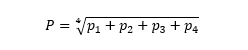在GIS里就是把数据弄进属性表里，然后用字段计算器用引力公式计算结果，最后分级表现。

要实现城市间的经济联系图，需要将每个城市分别作为起点和终点两两组合得到城市之间的连线，将每条线都附上城市之间引力值（通过公式求出的值）便能得到城市联系网络图。
那么要在ArcGIS里实现，需要做的事有两件。
每个城市的x,y坐标点  城市之间两两组合排列
之后使用xy转线工具，得到城市之间的联线。最后将计算出的引力值，将其连接至线上即可。

二、操作步骤
以2019年山东省16个地级市为例，选取GDP作为城市规模指标（单一，肯定不是很严谨，仅供参考）。Q为GDP，d为距离，a为常量，不影响，可取值为1。
将山东省16地市面要素转为点要素，在属性表里添加了xy字段，通过计算几何计算得出xy坐标。各市GDP数据连接进来。然后是数据的投影，不然计算距离用地理坐标系是不行的。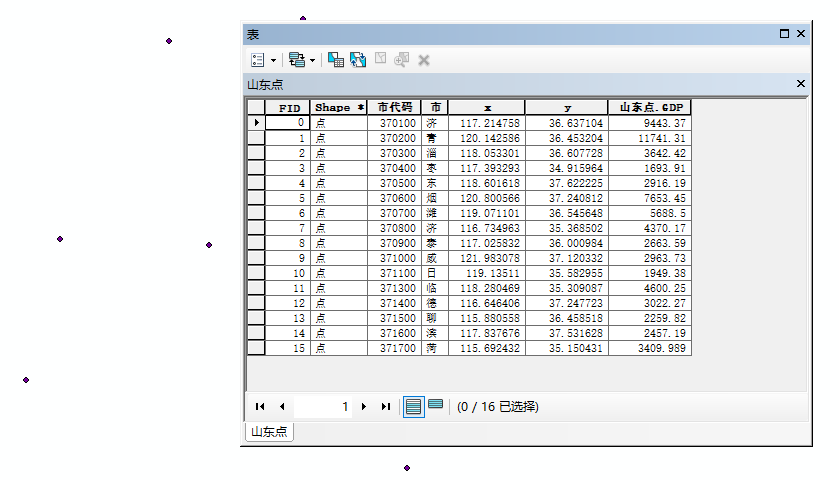用【点距离】工具来计算，注意这里输出表路径选择默认，我选择其他路径会报错，不知道什么原因。打开输出的表，每个FID都代表一个城市，input_FID相当于起点城市，near_FID就相当于终点城市，distance便是两个城市间的距离。
上表中的FID与下面这个表中的input_FID，near_FID是对应的。需要把它们关联起来，不然看数字谁知道是哪个城市。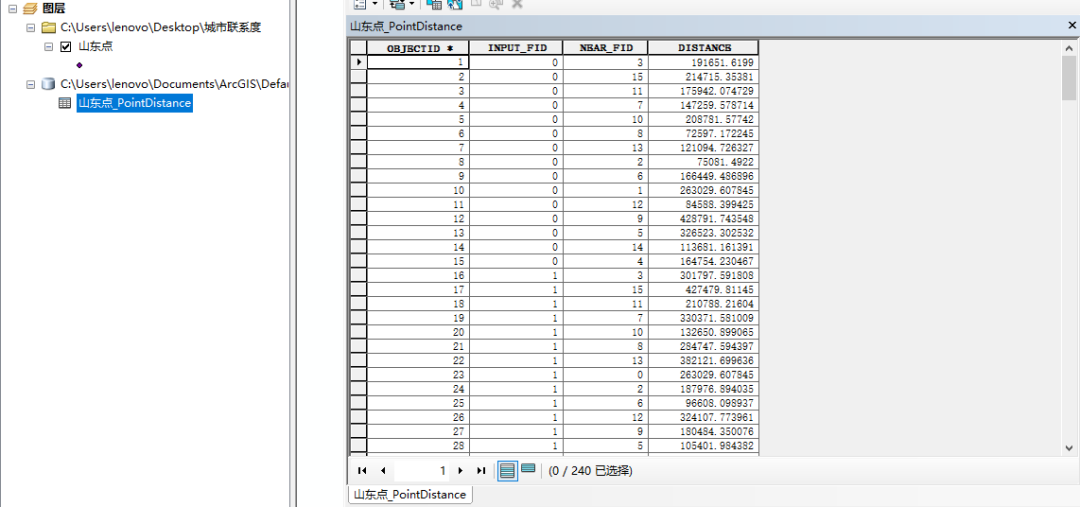可以在GIS里进行连接和关联，也可以直接复制到excle表格里实现。我jiao的用excle简单些，所以我选择excle来做。
复制上面两步的两个属性表到excle（Ctrl+A全选-右键FID左边空白-复制所选项），在excle里把用不着的字段删掉就可以。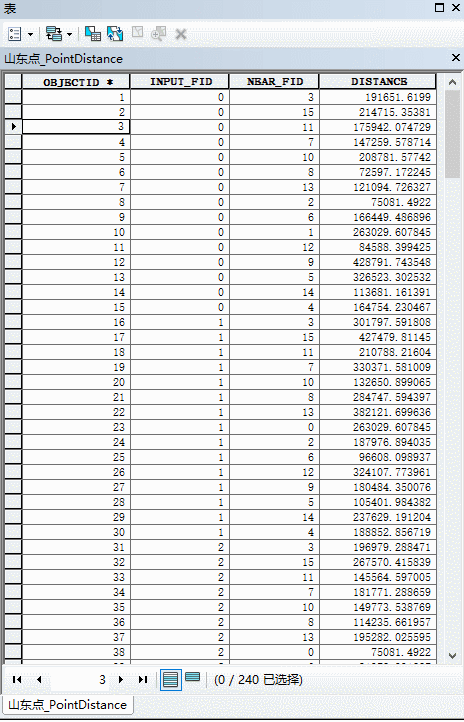复制出来得到这两个表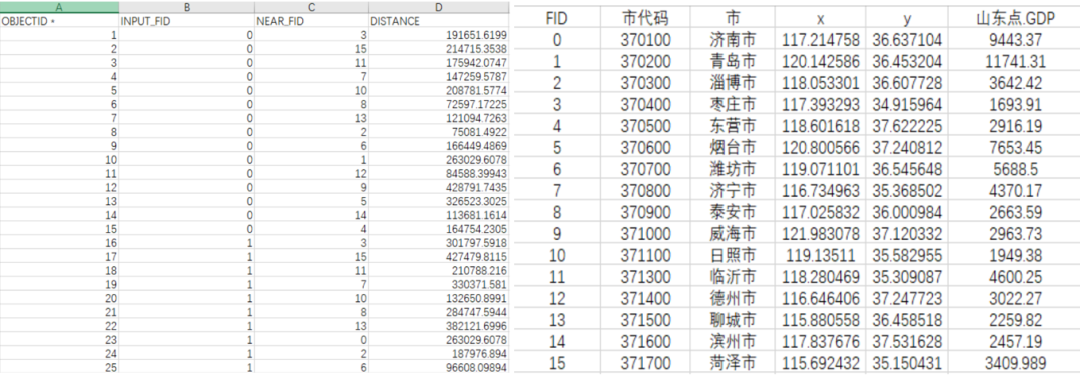在第一个表格里新增加6个表头，分别是or代表起点城市，ox、oy代表起点城市坐标；des代表终点城市，dx、dy代表终点城市坐标。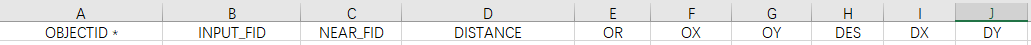接下来用到的就是excle里的vlookup函数了。简单说一下vlookup的意思：
Lookup_value是我们需要对应的那一列，先做的起点城市，先选input_fid。
Table_array是对应的哪个表，选中sheet2全部。
Col_index就是对应的sheet2表里的哪一列，城市名称是第三列，输入3即可。剩下的表头填充都是这方法，填充进去即可，不再演示。最后得到包含起点终点城市名，xy坐标，起终点GDP数据的表格。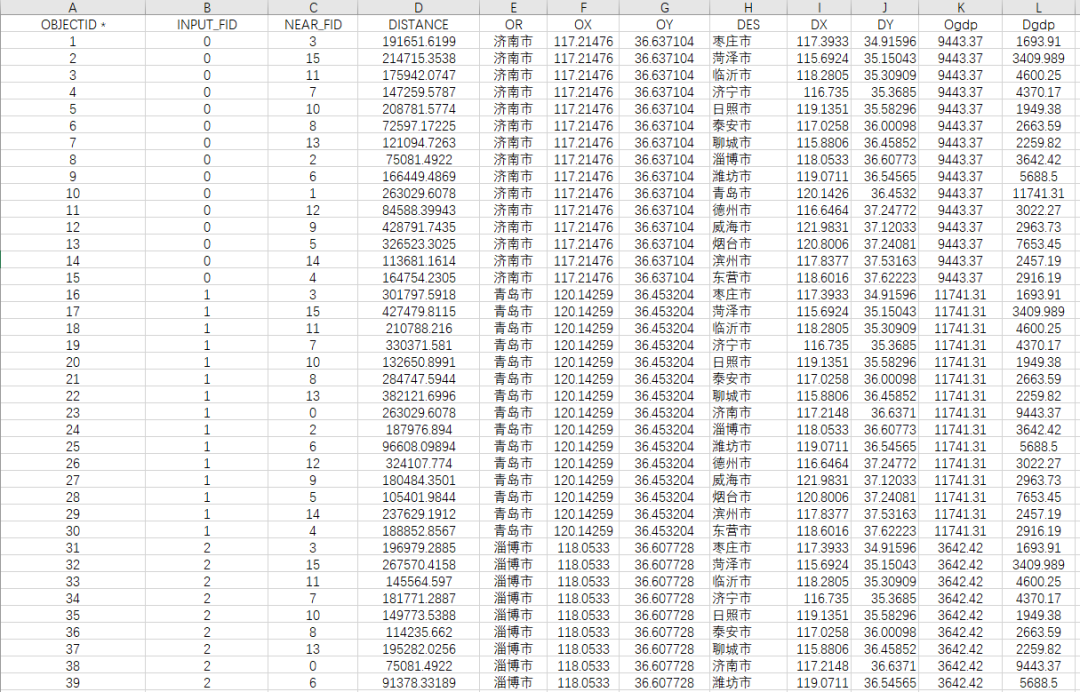接下来用开头的公式进行计算最开始计算的距离单位是米，这里把距离除1000，换算成千米。
Value（引力值）=ogdp（起点城市GDP）*dgdp(终点城市GDP)/距离的平方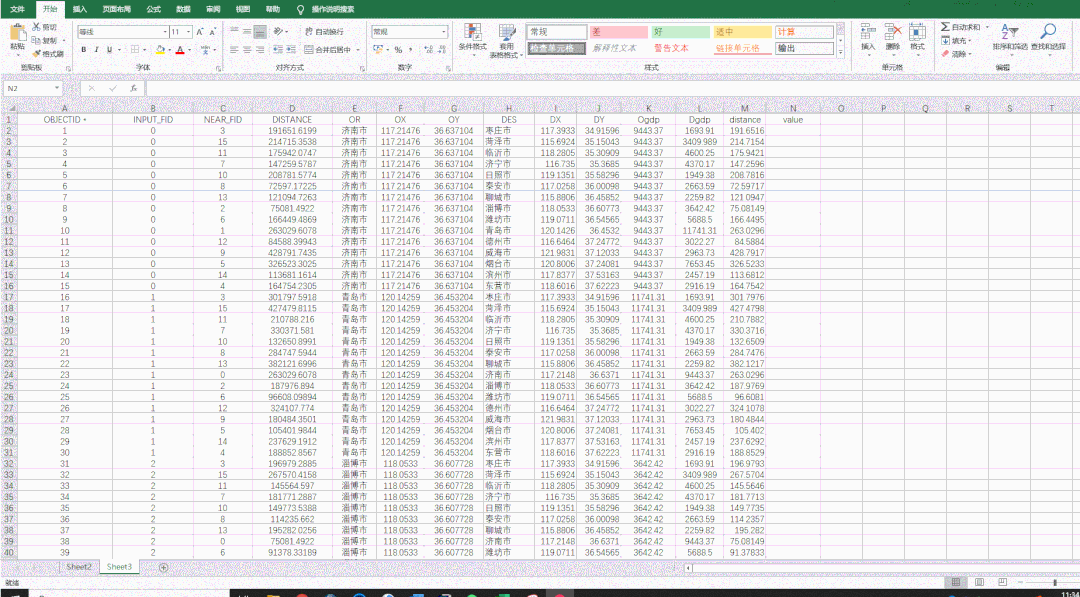打开ArcGIS里XY转线工具，依次输入选项，ID选择计算出来的引力值VALUE。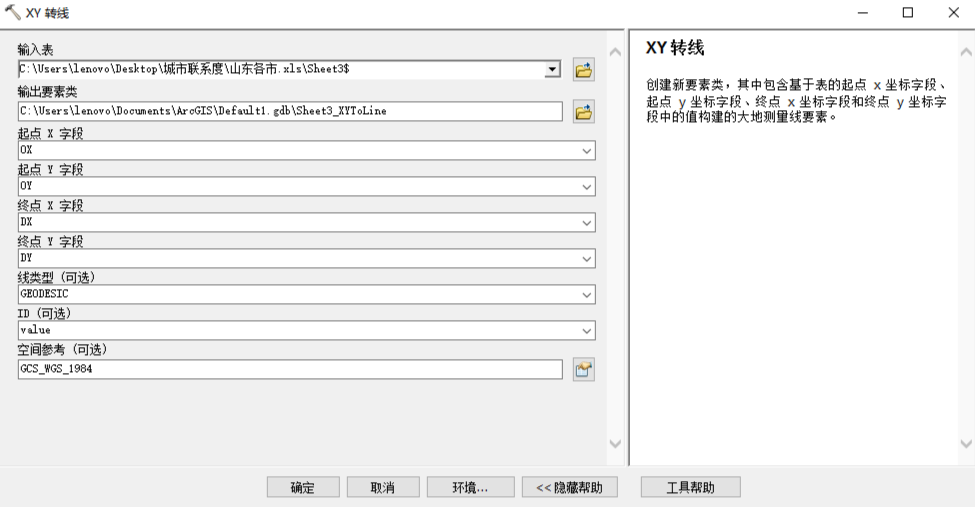这样我们初步的结果就出来了，可以进一步对其可视化。最后大致是这个样子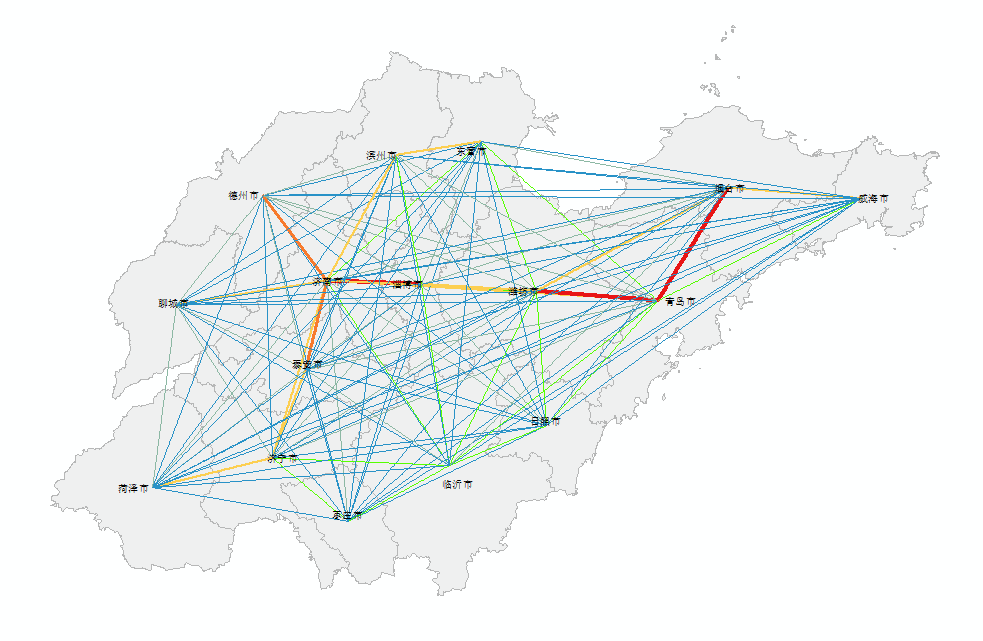Qgis作图比Arcgis的功能好多了，这次就放到Qgis里面试了一下，操作与Arcgis都是相通的，加载一下在线底图和图层文件，同样对线进行符号化显示就可以。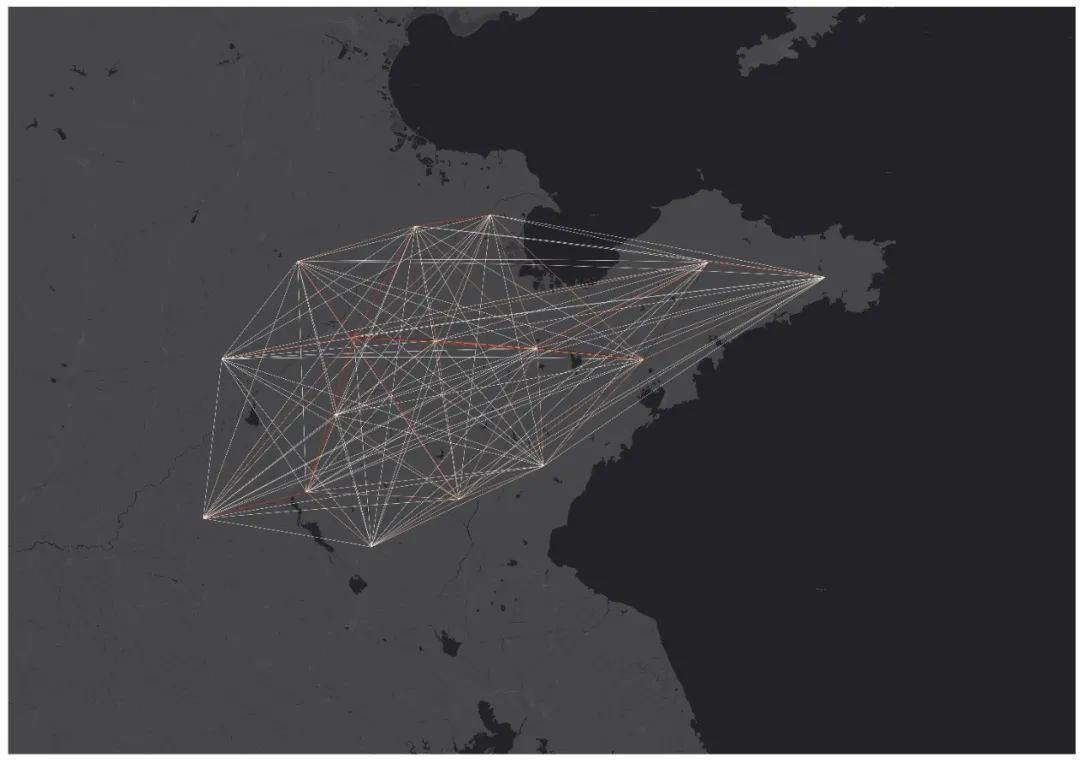可以看到，半岛地区，青岛与潍坊和烟台之间引力较大。西部就是济南与淄博，其次是德州和泰安。仅用了GDP这个单一指标进行试验，很难相对全面考察联系强度，初始重力模型太简单，也没去看很多论文再改进，如果仅用作平时项目分析之类的我觉得可以用用。

最后，Qgis加载在线底图的插件下载地址：
https://plugins.qgis.org/plugins/HCMGIS/
Arcgis还有一个OD插件工具可以把这种直线变为弧度线，我没试过，链接也放在这，有需自取：
https://pan.baidu.com/s/1UIuBIOWzVnPmrhXaZF7ggQ
提取码：c0es

END
如果在实验过程中有什么地方遇到问题可以加我，竭力解答。需要练习数据也请后台我，公主号【一点规划】。
如果可以的话，希望能够点个关注转发分享，点个在看并且点个赞~~

展开全文• matlab绘制引力场 您在一个街区。 有风，小雨正在下。 人们来回穿梭，携带着大量的粉笔和防水布。 许多人蹲在街上，他们的粉笔艺术受苦并诅咒着雨。 这条街向南延伸。 一条肮脏的混凝土楼梯井通向街道下方。 >...

matlab绘制引力场
您在一个街区。 有风，小雨正在下。 人们来回穿梭，携带着大量的粉笔和防水布。 许多人蹲在街上，他们的粉笔艺术受苦并诅咒着雨。 这条街向南延伸。 一条肮脏的混凝土楼梯井通向街道下方。

>下去
楼梯的底部是一扇敞开的门，通向一个黑暗的房间。 黑暗似乎从楼梯间底部的门口和游泳池溢出。 楼梯向上。 一个没有照明的门向东打开。
>往东走
您输入。 有了这么少的光线，您看不到多少东西。 您举起手，几乎无法辨认其形状。 当您抽出手时，远处墙壁上的一小束光突然爆炸，使您的视线陷入彩虹。欢迎来到DiMo
Digital Motion（DiMo）是GeekSpark的年度互动艺术展览，该展览每年在北卡罗来纳州罗利的SparkCon期间举行。
从表面上看，这是您挥舞手臂与巨大的投影屏进行交互的事件（今年，玩家挥舞着发光的警棍与计算机生成的图形进行交互）。 从更深层次上讲，GeekSpark的目标是使访客充满乐趣，然后使他们能够探索其背后的技术。 为此，DiMo背后的所有代码都是开源的。
2014年是我作为志愿者的第一年，与所有人一起工作是一次非常有趣的教育。 以下是制作这些展览品时团队合作的简短描述：
红帽工程师Ian Hands创建了一个光传感器系统，使玩家可以通过挥动警棍来与显示器互动。 共有三个可视化，所有这些可视化都是围绕光传感器系统构建的。 显示屏从一次可视化显示每五分钟旋转一次。
乔纳森·里皮（Jonathan Rippy）创作了《 康威的人生游戏 》的互动版本。Wray Bowling创建了一个有趣的三人游戏，结合了多力多滋，乡巴佬，几何和苏打水。我创建了一个粒子物理sim卡，允许玩家与重力场（和漂亮的颜色）进行交互。在探讨技术细节之前，请查看此现场演示 。
该演示使您可以使用鼠标而不是发光警棍来玩粒子模拟。 需要现代的网络浏览器，最好是Firefox或Chrome。 按空格键在播放器之间切换，然后使用设置面板滑块创建全新的可视化效果。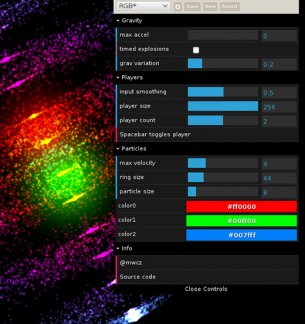DiMo的起源
去年五月，伊恩（Ian）与我联系，为GeekSpark做贡献。 经过一连串的休息室讨论和具有象征意义的餐巾素描后，我开始研究粒子模拟，而他正在研究传感器系统。 Jonathan和Wray从Red Hat外部慷慨地自愿捐款。
这个想法是：将发光的警棍散发给玩家，然后他们可以挥手与酷炫的墙面显示器互动。 工作原理：一台在天花板附近装有广角网络摄像头的计算机将在每个播放器的位置上保持标签，并将这些位置传递给可视化效果，从而使播放器部件在屏幕上移动。
DiMo的三种可视化效果是由HTML，CSS和JavaScript组成的常规网页。 之所以选择将Web用于此项目，是出于以下几个原因：
较低的进入壁垒是一个很大的障碍。 只需几分钟和少量指导，即使没有编程经验的人也可以开始自己做。 图形编程在所有类型的编程中都是独一无二的。 通常，方程式的意外变化（甚至是错字！）都会带来非常酷的惊喜。 “仅需尝试”就能获得比其他任何编程分支更大的收益。  活动结束后共享页面就像单击链接一样简单。 与其他平台相比，网络更容易将您的像素摆在人们面前。  Web本质上是开放的。 每个（桌面）网络浏览器都有一个“查看源代码”按钮，因此任何有意愿的人都可以找到代码。
接下来，DiMo 2015！
您想制作自己的DiMo显示器吗？ 保持联系！ 2015年DiMo活动的计划即将开始，因此没有比现在更好的参与时间了！ 对Raleigh而言是本地的，这很好，但是并不需要。 不过，如果您可以降低SparkCon的积分，您将获得加分。 看到所有陌生人都喜欢自己制作的东西，这无比喜悦。链接和其他好奇心：
传感器系统（输入）和粒子模拟（渲染器）的源代码  粒子模拟的视频  设置晚会的间隔拍摄视频  演示如何使用控件创建新效果的视频  有关粒子模拟的更多信息  Wray Bowling的图片组合

翻译自: https://opensource.com/life/15/2/sparkcon-geekspark-digital-motion-exhibit

matlab绘制引力场

展开全文可视化 python 数据可视化 人工智能
• 在惯性制导过程中需要时刻计算弹道点...重点研究了3种弹道扰动引力计算方法：地球重力场模型、点质量模型、单层密度模型，分别推导了其计算扰动引力的基本公式，并进行了数值模拟实验，分析比较了其精度和计算效率。
• c++代码，GSA 引力搜索算法详细执行步骤，可直接运行，有标注，是学习的好资料，欢迎下载
• 是地心引力常数，是地球自转角速率，其值的大小参考对应的ICD文档。 1、广播星历参数表 参考时间： 轨道长轴平方根： 偏心率： 近地点幅角： 卫星平均运动速率与计算值之差： 参考时刻平近点角： 参考时刻...
• 文章目录第三十一章 引力搜索算法（Gravitational Search Algorithm，GSA）万有引力定律GSA 第三十一章 引力搜索算法（Gravitational Search Algorithm，GSA） 2009，伊朗的Esmat Rashedi等人基于万有引力定律和粒子...智能优化
• 作为主要示例，我们显示了如何为完全相对论的经典单环贡献对于质量不同的两个粒子的散射获得一个紧凑的公式。 领先奇点的非相对论性极限与后牛顿扩张中的已知结果一致。 我们还计算了各种更高的循环前导奇点，包括...
• 对于自旋为0,1 / 2和1的粒子，我们通过重力an灭计算相关截面，并给出具有完全质量相关项的解析公式。 我们发现，质量在TeV和1016 GeV之间的DM可能具有适合观察的文物丰度，而对自旋的依赖性很小。 我们还讨论了来自...
• (3)分子间的引力和斥力同时存在,随分子间距离的增大而减小,但斥力减小得比引力快； (4)分子力做正功，分子势能减小,在r0处F引＝F斥且分子势能最小； (5)气体膨胀,外界对气体做负功W；温度升高，内能增大ΔU>0；吸收...
• 优化算法之引力搜索算法（gravitational search algorithm），主要是利用两物体之间的引力定律进行指导各个粒子的运动优化搜索最优解的，两个粒子之间的引力与两个粒子的质量成正比，与两粒子之间的距离成反比算法
• 以优化SVM算法的参数c和g为例，对GSA（引力搜索）算法MATLAB源码进行了详细中文注解。matlab 算法 优化
• §6.5 功、水压力和引力 一、变力沿直线所作的功 【例1】半径为的球沉入水中，球的上部与水面相切，球的比重为 1 ，现将这球从水中取出，需作多少功? 解：建立如图所示的坐标系 将高为的球缺取出水面，所需的...高等数学 高等数学
• ## 计算万有引力

千次阅读 2018-03-30 18:29:44
编写程序，计算两个星球之间的万有引力。 两个星球的质量m1,m2由用户从键盘输入，单位为kg... 万有引力的计算公式为：F=G*m1*m2/R/R程序的运行结果如下所示： 输入(三个数据依次为：m1, m2和R)：1.987E30 5.975...
• ## 改进型人工势场法

万次阅读 多人点赞 2019-06-14 09:39:34
引力计算相似，斥力的计算公式为： F r e p = − ∇ U r e p ( q ) = { 0 , if  ρ o b s ( q ) ≥ ρ 0 K r e p ( 1 ρ o b s ( q ) − 1 ρ 0 ) ( 1 ρ o b s 2 ( q ) ) q − q o b s ∣ ∣ q − q o b s ∣ ...人工势场法 路径规划
• 压强公式，压力公式： 压强 ： 公式：P=ρgh 式中g=9.8N/kg 或g=10N/kg,h的单位是m ,ρ的单位是kg/m^3;...两个可看作质点的物体之间的万有引力，可以用以下公式计算： （r是指两物之间的距离） ，即万有引...
• 在本文的第一部分，我们将蝶形速度公式推广为各向异性时空。 我们应用该公式来评估M形，D形和弦背景中的蝴蝶速度。 我们显示，M2-大脑，M5-大脑和... 我们使用此公式计算不同背景下的蝶形速度，并讨论相关的属性。
• 我们无条件地提出了针对特定类别的f（R）= R +αR2的一类电磁平面水平D维解，所有这些解都表现为反de-Sitter时空... 我们使用Komar公式计算守恒量。 我们研究奇点并计算霍金温度和熵，并证明始终满足热力学第一定律。
• (3)分子间的引力和斥力同时存在,随分子间距离的增大而减小,但斥力减小得比引力快； (4)分子力做正功，分子势能减小,在r0处F引＝F斥且分子势能最小； (5)气体膨胀,外界对气体做负功W；温度升高，内能增大ΔU>0；吸收...
• 英文：(Gravitational wave)，台湾学界称为重力波，英文中有时也写作 gravity wave；...相对论中，爱因斯坦则认为是一种跟电磁波一样的波动，称为引力波。引力波是时空曲率的扰动以行进波的形式向外传递。引...
• 10分钟，搞懂引力波 https://baijiahao.baidu.com/s?id=1581560461270739279&wfr=spider&for=pc ...昨天晚上10点，全球数十家著名天文机构同时发布了一个重大消息：人类第...可能有人会说，引力波不是去...
• 在理想状态情况下，地球、卫星都是一个均匀的理想球体，且卫星只受地球引力影响，GPS卫星运行轨道为无摄运行轨道。通常，人们广泛使用开普勒轨道参数对GPS卫星无摄运行轨道进行描述。 以地球地心O为原点，建立如图1...GPS轨道
• 本文将介绍将卫星从地球发送到火星的仿真结果和分析。 我们使用Python模拟火箭的轨道。 我们的目标是找到最小的... 考虑到太阳，地球和火星的引力，我们根据霍曼转移轨道找到了允许卫星从地球到火星最短距离的日期。...